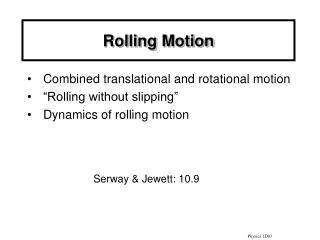DownloadDownload PresentationRolling Motion

# Rolling Motion

Télécharger la présentation## Rolling Motion

- - - - - - - - - - - - - - - - - - - - - - - - - - - E N D - - - - - - - - - - - - - - - - - - - - - - - - - - -
##### Presentation Transcript

1. Rolling Motion • Combined translational and rotational motion • “Rolling without slipping” • Dynamics of rolling motion Serway & Jewett: 10.9 Physics 1D03

2. General Motion of a Rigid Body Gives linear acceleration of the position of the center of mass. Gives angular acceleration of the body about the center of mass. Kinetic energy: These are special properties of the centre of massonly (although t = Ia is also true for any stationary axis of rotation). Physics 1D03

3. Rolling Motion w Different points on a rolling object have different velocities (blue vectors). The velocity of each particle(blue) is the velocity of the centre(red) plus the velocity rwrelative to the centre(green): v= vCM+ rω C vC The point in contact with the ground is momentarily stationary. The point on the top of the wheel moves forward at twice the speed of the centre. Physics 1D03

4. q R s = Rq If the body rolls without slipping, then its angular velocity and angular acceleration are related to the linear velocity and tangential acceleration of the centre: v = Rw anda = Ra Proof: When the object turns through an angle q, it moves forward a distance s = Rq (if it doesn’t slip). s = Rq; take derivatives wrt t to get: vcentre = Rw andacentre = Ra Physics 1D03

5. Quiz There is friction between a wheel and the road, otherwise a car could not accelerate. This friction is what causes a rolling wheel to stop. a) True b) False Physics 1D03

6. Friction and Rolling Resistance Friction is necessary to create a torque which can cause rotation and rolling. pull R f This is static friction if the wheel rolls without slipping. At the point of contact between the road and wheel, there is no relative motion, and friction does no work. “Rolling resistance” which slows the wheel results from the tire (and the road surface) flexing inelastically at the contact point. This can be a small effect, so wheels roll a long way. Physics 1D03

7. these are related by the condition for rolling CM motion: Rotation about CM: 2. Energy: Use Translational energy from CM: Rotational energy about CM axis: These are related by the condition for rolling Symmetrical rolling objects (CM in centre): 1. Dynamics: Use Physics 1D03

8. Example: A cylinder (mass m, radius R) rolls down a ramp without slipping. What is its speed after it has descended a vertical height h? n f h mg Which forces do work? Physics 1D03

9. y N f a x mg How would you calculate the force of static friction? forces (x components): mg sin q – f = maCM torques (clockwise): Rf = ICM a rolling: aCM= Ra solve (exercise for student!) ... Physics 1D03

10. Example: A solid cylinder and a hollow pipe roll down a ramp without slipping. a) Which gets to the bottom first? b) If they have equal masses, which has the greatest kinetic energy at the bottom? c) If the surface were slippery, would the time increase or decrease? d) For a moderate coefficient of friction, which can roll down the steepest slope without slipping? Physics 1D03

11. y N f a x mg d) Icyl = ½ mR2Ipipe = mR2 So f is larger if I is larger. So the pipe needs more friction than the solid cylinder. Physics 1D03

12. The general motion of a rigid body (i.e., not pure rotation about a fixed axis) can be described as translation of the center of mass, plus rotation about the center of mass. vCM  Kinematics CM Refer to motion of center of mass aCM = g Refer to rotation about an axis though the center of mass, which travels with the center of mass Physics 1D03

13. Quiz On a dry day, a round steel pipe is released from rest in a concrete dainage ditch. It rolls down without slipping, and then rolls up the other side to almost its original height. On a rainy day, there is less friction, and the pipe slips partially on the way down before it rolls. How far up the other side will it go? • Just as high as on the dry day. • A little higher. • Not as high. Physics 1D03

14. Summary For arbitrary motion of a rigid body, divide the motion into a linear motion of the centre of mass, plus rotation about the centre of mass. Then: Rolling without slipping: v = R w at = Ra Practice problems: 11-5, 11-47, 11-63 Physics 1D03